# Water Images- Test 4

1)

Choose the correct water image of the question figure, from the given answer figures (assume that water is along XY)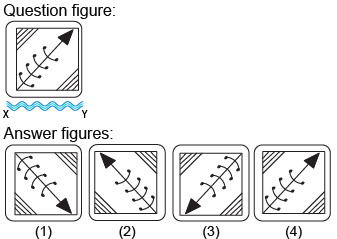2)

Choose the correct water image of the question figure, from the given answer figures (assume that water is along XY)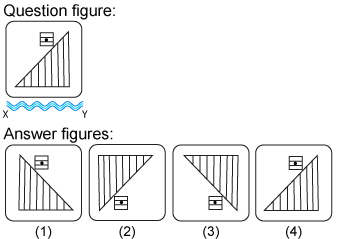3)

Choose the correct water image of the question figure, from the given answer figures (assume that water is along XY)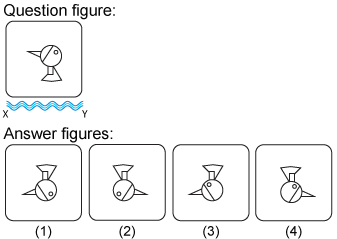4)

Choose the correct water image of the question figure, from the given answer figures (assume that water is along XY)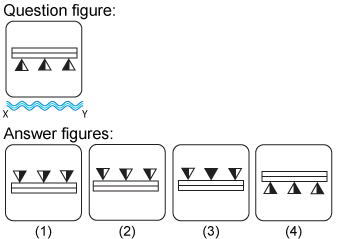5)

Choose the correct water image of the question figure, from the given answer figures (assume that water is along XY)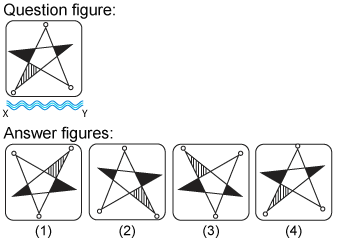--Share with your friends --